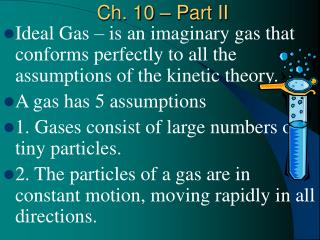DownloadDownload PresentationCh. 10 – Part II

# Ch. 10 – Part II

Download Presentation## Ch. 10 – Part II

- - - - - - - - - - - - - - - - - - - - - - - - - - - E N D - - - - - - - - - - - - - - - - - - - - - - - - - - -
##### Presentation Transcript

1. Ch. 10 – Part II • Ideal Gas – is an imaginary gas that conforms perfectly to all the assumptions of the kinetic theory. • A gas has 5 assumptions • 1. Gases consist of large numbers of tiny particles. • 2. The particles of a gas are in constant motion, moving rapidly in all directions.

2. 3. The average kinetic energy of the particles of a gas is directly proportional to the temp. of a gas. • KE= ½ MV • 4. There are no forces of attraction or repulsion between the particles of a gas. • 5. The collision between particles of a gas and between particles and container walls are elastic collisions.

3. Fluid – a gas or liquid • A gas is about 1/1000 the density of the same substance as a solid or liquid. Why? • Molecules are farther apart. • Compression – gas under pressure. • By compressing a gas you can have as much as 100 times more molecules in a cylinder than uncompressed. • Effusion – is a process by which gas particles under pressure pass through a very small opening from one container to another. • What is diffusion?

4. Real gas – is a gas that does not behave completely according to the assumptions of the kinetic energy. • Johannes van der Waals proposed this. • Real gases are explained by the following: • 1. Particles of real gases occupy space • 2. Particles of real gases exert attractive forces on each other. • Gases behave different when heated, cooled, or under pressure. • Under “normal conditions” a gas is considered to be an ideal gas.

5. Gases have 4 measurable quantities • 1. Volume • 2. Pressure • 3. Temperature • 4. Quantity of molecules (number) • If 3 of these quantities are known then you can figure the fourth one.

6. If air is heated it can expand its volume many times. If it’s cooled it compresses. • Pressure is measured by how fast the gas molecules are moving. Determined by how many times the molecules hit the container it is in. • Ex. Small vs. large container with 10 molecules. • Temp. increases = pressure increase • Temp. decreases = pressure decrease • Volume decreases = pressure increase • Volume increase = pressure decrease

7. The more molecules of gas in a container, the more pressure it has. Why? • In the winter time the pressure in your car tire is less. Why? • If you blow up a balloon the volume is constant if the temp. and pressure are constant. • IDEAL GAS LAW CONSTANT • R = PV/nT

8. Pressure – is the force per unit area on a surface • P = f/a • Label  N/cm2 or Pascals • 1 N/cm2 = 1 Pascal • The SI unit for force is Newtons (N) • Barometer – is a device used to measure the atmospheric pressure. • Torricelli discovered this. • In a vacuum condition and a sea level a colum of mercury or barometer will rise 760 mm.

9. 760 mm of Hg is the atmospheric pressure at sea level and at 0 degrees C. • 760 mm of Hg = 760 torr. or 1 atm. of pressure • Sample Problem 10.1 • Standard conditions or STP – standard temperature and pressure. • 1 atm. Of pressure, 760 torr., or 760 mm Hg  Pressure • 273 K or 0 degrees Celsius  Temperature • 1 Liter or 1000 ml  Volume

10. Robert Boyle discovered that pressure and volume are inversely proportional to each other. • Ex. Double the volume = ½ the pressure • Ex. Triple the pressure = 1/3 the volume • Ex. Pushing in on the sides of a balloon increases the pressure of the air inside the balloon. • P1V1 = P2V2 • Sample Problem 10-2

11. Charles’ Law – states that the volume of a gas varies directly to the temperature of the gas. • Ex. Double the temp. = double the volume • Ex. Hot air balloon • V1/T1 = V2/T2 • Sample Problem 10-3

12. Gay-Lussac’s Law – states that the pressure of a gas is directly proportional to the temp. of the gas. • Ex. Double temp. = double the pressure • Ex. Car tires • P1/T1 = P2/T2 • Sample Problem 10-4

13. Combined gas law – shows the relationship between pressure, volume, and temp. of a gas when the amount of gas is fixed. • P1V1/T1 = P2V2/T2 • Sample Problems